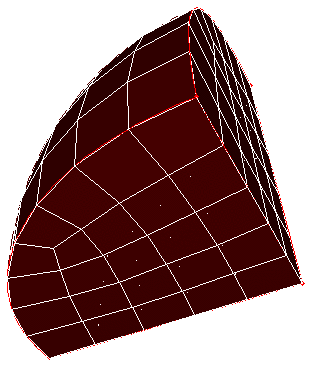# Tetprimitive

Applies to: Volumes

Summary: Meshes a 4 "sided" object with hexahedral elements using the standard tetrahedron primitive.

Syntax:

Volume <range> Scheme Tetprimitive [Combine Surface <range>] [Combine Surface <range>] [Combine Surface <range>] [Combine Surface <range>]

Discussion:

The tetprimitive scheme is used to create a hexahedral mesh in a volume which fits the shape of a tetrahedral primitive. The Tetprimitive scheme assumes that each of the four surfaces have been meshed with the triprimitive, or similar, meshing scheme. If more than four surfaces form the tetrahedron geometry, the surfaces forming a logical side can be combined using the combine option.Figure 1. Sphere octant hex meshed with scheme Tetprimitive, surfaces meshed using scheme Triprimitive